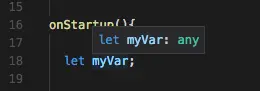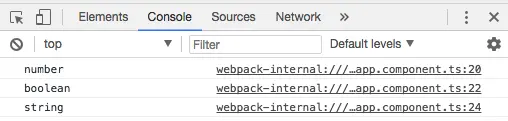# Typescript variable types

### Type: any

You could ask — what if I don't know what kind of value my variable will have?

1. Let's flush our function code and type ine the following:
2. ``````  let myVar;

myVar = 1;      //number
myVar = true;   //boolean
myVar = 'mdb';  //string
``````

The TypeScript compiler doesn't complain. Why!?

3. Hover over the `myVar` declaration, what do you see?
4.TypeScript allows you to use a special type `any`. It allows you to use dynamic typing but importantly — it requires you to use it consciously.

5. What is actually type of the variable `myVar`? Let's check it:
6. ``````  myVar = 1;     //number
console.log(typeof(myVar));
myVar = true;  //boolean
console.log(typeof(myVar));
myVar = 'mdb'; //string
console.log(typeof(myVar));
``````

The output:7. Now let's add an explicit type, cast after the var declaration:
8. ``  let myVar: number;``

Immediately, the compiler will show two errors regarding the second, and third assignments### Other types:

We used already four different types of variables (`number`, `string`, `boolean` and `any`). Are there any others? Yes, there are.

``````  let a: number;                    //numeric type
let b: string;                    //string
let c: boolean;                   //true or false
let d: any;                       //any (dynamic)
let e: number[] = [1,2,3];        //array of numbers
let f: string[] = ['a','b','c'];  //array of strings
let g: any[] = [true, 1, 'a'];    //array of any
``````

As you can see, except for basic types which we already covered you can also create arrays of different types including the `any` type. There is also one more type of variable — `enum` but we will cover it in the future.